# HSSlive: Plus One & Plus Two Notes & Solutions for Kerala State Board

## AP Board Class 7 Maths Chapter 11 Exponents InText Questions Textbook Solutions PDF: Download Andhra Pradesh Board STD 7th Maths Chapter 11 Exponents InText Questions Book AnswersAP Board Class 7 Maths Chapter 11 Exponents InText Questions Textbook Solutions PDF: Download Andhra Pradesh Board STD 7th Maths Chapter 11 Exponents InText Questions Book Answers

## Andhra Pradesh State Board Class 7th Maths Chapter 11 Exponents InText Questions Books Solutions

 Board AP Board Materials Textbook Solutions/Guide Format DOC/PDF Class 7th Subject Maths Chapters Maths Chapter 11 Exponents InText Questions Provider Hsslive

2. Click on the Andhra Pradesh Board Class 7th Maths Chapter 11 Exponents InText Questions Answers.
3. Look for your Andhra Pradesh Board STD 7th Maths Chapter 11 Exponents InText Questions Textbooks PDF.

## AP Board Class 7th Maths Chapter 11 Exponents InText Questions Textbooks Solutions with Answer PDF Download

Find below the list of all AP Board Class 7th Maths Chapter 11 Exponents InText Questions Textbook Solutions for PDF’s for you to download and prepare for the upcoming exams:

Do This

Question 1.
Write the following in exponential form, (values are rounded off) (Page No. 212)
i) Total surface area of the Earth is 510,000,000 square kilometers.
Solution:
51 × 107 = 3× 17 × 107

ii) Population of Rajasthan is approximately 7,00,00,000.
Solution:
7 × 107

iii) The approximate age of the Earth is 4550 million years.
Solution:
4550 millions = 4550 × 10,00,000 (v 1 million =10 lakhs)
= 455 × 107 = 91 × 5 × 107 = 5 × 7 × 13 × 107

iv) 1000 km in meters.
Solution:
1 km = 1000 m
∴ 1000 km = 1000 × 1000 m = 106

Question 2.
Express (i) 48951 (ii) 89325 in expanded form using exponents. (Page No. 212)
Solution:
i) 48951 = (4 × 10000) + (8 × 1000) + (9 × 100) + (5 × 10) + (1 × 1)
= (4 × 104) + (8× 103) + (9 × 102) + (5 × 1.0) + (1 × 1)

ii) 89325 = (8 × 10000) + (9 × 1000) + (3 × 100) + (2 × 10) + (5 × 1)
= (8 × 104) + (9 × 103) + (3 × 102) + (2 × 10) + (5 × 1)

Question 3.
Is 32 equal to 23 ? Justify. (Page No. 213)
Solution:
32 ≠ 23
Since 32 = 3 × 3 = 9 and 23 = 8
∴ 32 ≠ 23

Question 4.
Write the following numbers in exponential form. Also state the
a) base b) exponent and c) how it is read.
i) 32 ii) 64 iii) 256 iv) 243 v) 49 (Page No. 213)
Solution:
i) 32 = 2 × 2 × 2 × 2 × 2 = 25
Base = 2; exponent = 5; read as 2 raised to the power 5.
ii) 64 = 2 × 2× 2 × 2 × 2 × 2 = 26
Base = 2; exponent = 6 and we read it as 2 raised to the power 6.
iii) 256 = 2 × 2 × 2 × 2 × 2 × 2 × 2 × 2 = 28
Base = 2, exponent = 8 and we read it as 2 raised to the power 8.
iv) 243 = 3 × 3 × 3 × 3 × 3 = 35
Base = 3; exponent = 5 and we read it as 3 raised to the power 5.
v) 49 = 7 × 7 = 72
= 7 is the base ; exponent = 2.

Question 5.
Write the expanded form of the following. (Page No. 213)
i) p7 ii) l4 iii) s9 iv) d6 v) z5
Solution:
i) p7 = p × p × p × p × p × p × p
ii) l4 = l × l × l × l
iii) s9 = s × s × s × s × s × s × s × s × s
iv) d6 = d × d × d × d × d × d
v) z5 = z × z × z × z × z

Question 6.
Write the following in exponential form. (Page No. 213)
i) a × a × a × ………………….l’ times
ii) 5 × 5 × 5 × 5 × ……………..’n’ times
iii) q × q × q × q × q ………………….15 times
iv) r × r × r × ………………….’b’ times
Solution:
i) a × a × a × ………………….’l’ times = al
ii) 5 × 5 × 5 × 5 × ……………..’n’ times = 5n
iii) q × q × q × q × q …………….15 times = q15
iv) r × r × r × ……………..’b’ times = rb

Do This

Question 1.
Find the values of 24, 23 and 27 and verify whether 24 × 23 = 27. (Page No. 215)
24 = 2 × 2 × 2 ×2 = 16;
23 = 2 × 2 x 2 = 8
27 = 2 × 2 × 2 × 2 × 2 × 2 × 2 = 128
24 × 23 = 16 × 8 = 128 = 27
24 × 23 = 27

Question 2.
Find the values of 52, 53 and 55 and verify whether 52 × 53 = 55. (Page No. 215)
Solution:
52 = 5 × 5 = 25;
53 = 5 × 5 × 5 = 125 and 55 = 5 × 5 × 5 × 5 × 5 = 3125
Now 52 × 53 = 25 × 125 = 3125 = 55
∴ 52 × 53 = 55

Question 3.
Simplify the following using the formula am × an = am + n (Page No. 216)
i) 311 × 39 ii) p5 × p8
Solution:
i) 311 × 39 = 311+9 = 320
ii) p5 × p8 = p5+8 = p13

Question 4.
Find the appropriate number in place of the symbol’?’in the following. (Page No. 216)
Let ‘k’ be any non-zero integer.
i) k3 × k4 = k?
Solution:
i) k3 × k4 = k?
as k3 × k4 = k3+4 = k7 the value of ‘?’ = 7

ii) k15 × k? = k31
as k15 × k? = k15+?
but k15 + ? = k31
Since bases are equal we equate the exponents
∴ 15 + ? = 31
(i.e„) ? = 31 – 15 = 16

Question 5.
Compute 36, cube of 32 and verify whether (32)3 = 36. (Page No. 216)
Solution:
36 = 3 × 3 × 3 × 3 × 3 × 3 = 729.
cube of 32 = (32)3 = 93 = 9 × 9 × 9 = 729
Now (32)3 = 32 × 32 × 32 = 9 × 9 × 9 = 729
36 = 3 × 3 × 3 × 3 × 3 × 3 = 9 × 9 × 9
(32)3 = 36

Question 6.
Simplify the following using the law am × bm = (ab)m (Page No. 218)
i) (2 × 3)4
ii) xp × yp
iii) a8 × b8
iv) (5 × 4)11
Solultion:
i) (2 × 3)4 = 24 × 3 4 = (2 × 2 × 2 × 2) × (3 × 3 × 3 ×3) = 16 × 81 = 1296
ii) xp × yp = (x . y)p
iii) a8 × b8 = (a.b)8
iv) (5 × 4)11 = 511 × 411 = 511 × (2 × 2)11
= 511 × 211 × 211 = (5 × 2)11 × 211 = 1011 × 211

Question 7.
Write the following, by using 𝐚−𝑛=1𝐚𝑛 with positive exponents. (Page No. 219)
Solution: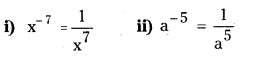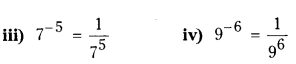Question 8.
Simplify and write in the form of am-n or 1𝐚𝐧−𝐦
i) 138135
ii) 34314
Solution: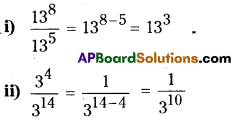Question 9.
Fill the appropriate number in the box. (Page No. 222)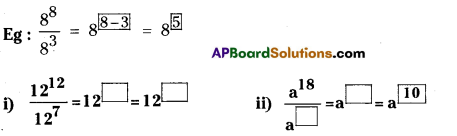Solution: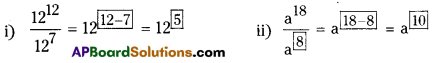Question 10.
Complete the following (Page No. 223)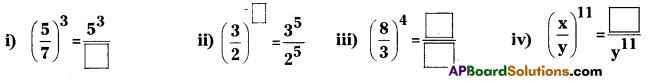Solution: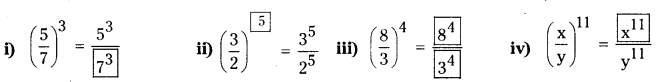Question 11.
Write in expanded form. (Page No. 224)
i) a-5
ii) (-a)4
iii) (-7)-5
iv) (-a)m
Solution: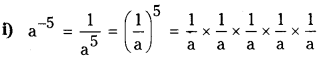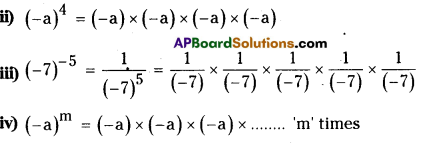Question 12.
Write in exponential form. (Page No. 224)
i) (-3) × (-3) × (-3)
ii) (-b) × (-b) × (-b) × (-b)
iii) (1−2)×(1−2)×(1−2) ………………….’m’ times
Solution:
i) (-3) × (-3) × (-3) = (-3)3
ii) (-b) × (-b) × (-b) × (-b) = (-b)4
iii) (1−2)×(1−2)×(1−2) ………………….’m’ times = (−12)𝑚 or (-2)-m

Do This

Question 1.
Write the following in exponential form using prime factorization. (Page – 214)
i) 2500 ii) 1296 iii) 8000 iv)6300
Solution:
i) 2500 = 2 × 1250 = 2 × 2 × 625
= (2 × 2) × 5 × 125
= (2 × 2) × 5 × 5 × 25
= (2 × 2) × (5 × 5 × 5 × 5)
= 22 × 54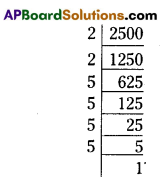ii) 1296 = 2 × 648 = 2 × 2 × 324 = 2 × 2 × 2 × 162
= (2 × 2 × 2 × 2) × 81
= (2 × 2 × 2 × 2 ) × 3 × 27
= (2 × 2 × 2 × 2 ) × 3 × 3 × 9
= (2 × 2 × 2 × 2 ) × ( 3 × 3 × 3 × 3 )
= 24 × 34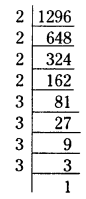iii) 8000 = 2 × 4000 = 2 × 2 × 2000 = 2 × 2 × 2 × 1000
= 2 × 2 × 2 × 2 × 500
= 2 × 2 × 2 × 2 × 2 × 250
= (2 × 2 × 2 × 2 × 2 × 2) × 125
= (2 × 2 × 2 × 2 × 2 × 2) × 5 × 25
= (2 × 2 × 2 × 2 × 2 × 2) × ( 5 × 5 × 5)
= (26 × 53)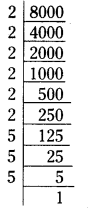iv) 6300 = 2 × 3150 = 2 × 2 × 1575
= (2 × 2) × 3 × 525
= 2 × 2 × 3 × 3 × 175
= (2 × 2) × (3 × 3) × 5 × 35
= (2 × 2) × (3 × 3) × (5 × 5) × 7
= 22 × 32 × 52 × 7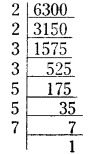## Andhra Pradesh Board Class 7th Maths Chapter 11 Exponents InText Questions Textbooks for Exam Preparations

Andhra Pradesh Board Class 7th Maths Chapter 11 Exponents InText Questions Textbook Solutions can be of great help in your Andhra Pradesh Board Class 7th Maths Chapter 11 Exponents InText Questions exam preparation. The AP Board STD 7th Maths Chapter 11 Exponents InText Questions Textbooks study material, used with the English medium textbooks, can help you complete the entire Class 7th Maths Chapter 11 Exponents InText Questions Books State Board syllabus with maximum efficiency.

## FAQs Regarding Andhra Pradesh Board Class 7th Maths Chapter 11 Exponents InText Questions Textbook Solutions

#### Can we get a Andhra Pradesh State Board Book PDF for all Classes?

Yes you can get Andhra Pradesh Board Text Book PDF for all classes using the links provided in the above article.

## Important Terms

Andhra Pradesh Board Class 7th Maths Chapter 11 Exponents InText Questions, AP Board Class 7th Maths Chapter 11 Exponents InText Questions Textbooks, Andhra Pradesh State Board Class 7th Maths Chapter 11 Exponents InText Questions, Andhra Pradesh State Board Class 7th Maths Chapter 11 Exponents InText Questions Textbook solutions, AP Board Class 7th Maths Chapter 11 Exponents InText Questions Textbooks Solutions, Andhra Pradesh Board STD 7th Maths Chapter 11 Exponents InText Questions, AP Board STD 7th Maths Chapter 11 Exponents InText Questions Textbooks, Andhra Pradesh State Board STD 7th Maths Chapter 11 Exponents InText Questions, Andhra Pradesh State Board STD 7th Maths Chapter 11 Exponents InText Questions Textbook solutions, AP Board STD 7th Maths Chapter 11 Exponents InText Questions Textbooks Solutions,
Share: